Documentation

# fir1

Window-based FIR filter design

## Syntax

``b = fir1(n,Wn)``
``b = fir1(n,Wn,ftype)``
``b = fir1(___,window)``
``b = fir1(___,scaleopt)``

## Description

example

````b = fir1(n,Wn)` uses a Hamming window to design an `n`th-order lowpass, bandpass, or multiband FIR filter with linear phase. The filter type depends on the number of elements of `Wn`.```

example

````b = fir1(n,Wn,ftype)` designs a lowpass, highpass, bandpass, bandstop, or multiband filter, depending on the value of `ftype` and the number of elements of `Wn`.```

example

````b = fir1(___,window)` designs the filter using the vector specified in `window` and any of the arguments from previous syntaxes.```
````b = fir1(___,scaleopt)` additionally specifies whether or not the magnitude response of the filter is normalized.Note:   Use `fir2` for windowed filters with arbitrary frequency response.```

## Examples

collapse all

Design a 48th-order FIR bandpass filter with passband $0.35\pi \le \omega \le 0.65\pi$ rad/sample. Visualize its magnitude and phase responses.

```b = fir1(48,[0.35 0.65]); freqz(b,1,512)```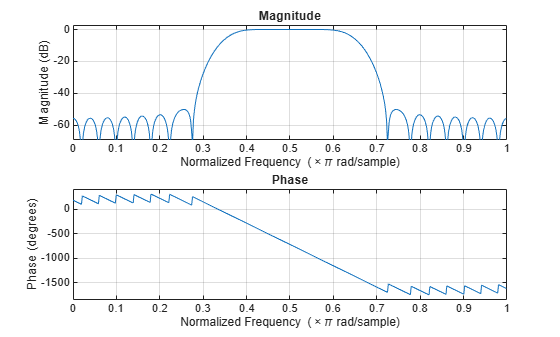Load `chirp.mat`. The file contains a signal, `y`, that has most of its power above `Fs/4`, or half the Nyquist frequency. The sample rate is 8192 Hz.

Design a 34th-order FIR highpass filter to attenuate the components of the signal below `Fs/4`. Use a cutoff frequency of 0.48 and a Chebyshev window with 30 dB of ripple.

```load chirp t = (0:length(y)-1)/Fs; bhi = fir1(34,0.48,'high',chebwin(35,30)); freqz(bhi,1) ```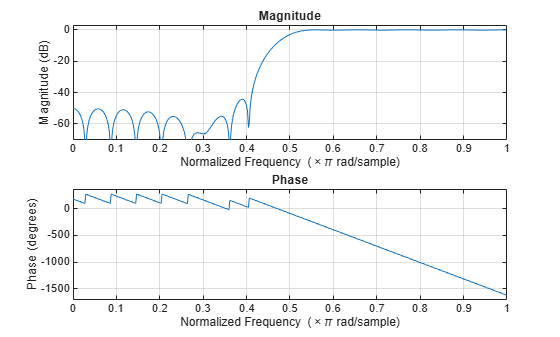Filter the signal. Display the original and highpass-filtered signals. Use the same y-axis scale for both plots.

```outhi = filter(bhi,1,y); subplot(2,1,1) plot(t,y) title('Original Signal') ys = ylim; subplot(2,1,2) plot(t,outhi) title('Highpass Filtered Signal') xlabel('Time (s)') ylim(ys) ```Design a lowpass filter with the same specifications. Filter the signal and compare the result to the original. Use the same y-axis scale for both plots.

```blo = fir1(34,0.48,chebwin(35,30)); outlo = filter(blo,1,y); subplot(2,1,1) plot(t,y) title('Original Signal') ys = ylim; subplot(2,1,2) plot(t,outlo) title('Lowpass Filtered Signal') xlabel('Time (s)') ylim(ys) ```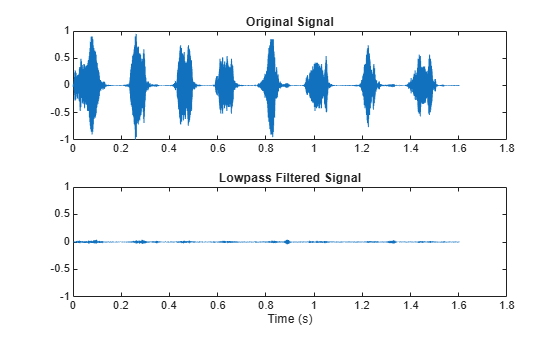Design a 46th-order FIR filter that attenuates normalized frequencies below $0.4\pi$ rad/sample and between $0.6\pi$ and $0.9\pi$ rad/sample. Call it `bM`.

```ord = 46; low = 0.4; bnd = [0.6 0.9]; bM = fir1(ord,[low bnd]);```

Redesign `bM` so that it passes the bands it was attenuating and stops the other frequencies. Call the new filter `bW`. Use `fvtool` to display the frequency responses of the filters.

```bW = fir1(ord,[low bnd],'DC-1'); hfvt = fvtool(bM,1,bW,1); legend(hfvt,'bM','bW')```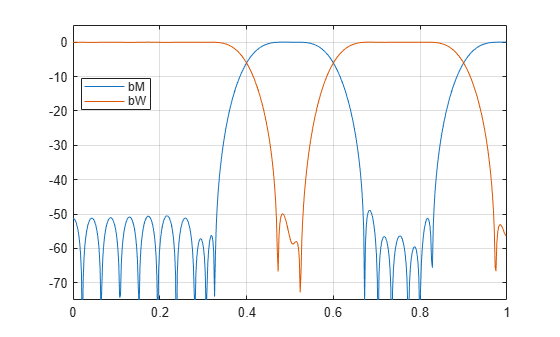Redesign `bM` using a Hann window. (The `'DC-0'` is optional.) Compare the magnitude responses of the Hamming and Hann designs.

```hM = fir1(ord,[low bnd],'DC-0',hann(ord+1)); hfvt = fvtool(bM,1,hM,1); legend(hfvt,'Hamming','Hann')```Redesign `bW` using a Tukey window. Compare the magnitude responses of the Hamming and Tukey designs.

```tW = fir1(ord,[low bnd],'DC-1',tukeywin(ord+1)); hfvt = fvtool(bW,1,tW,1); legend(hfvt,'Hamming','Tukey')```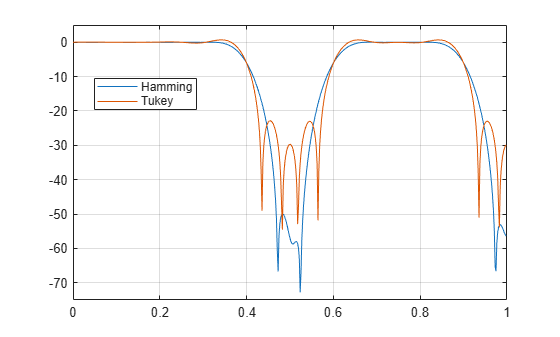## Input Arguments

collapse all

Filter order, specified as an integer scalar.

For highpass and bandstop configurations, `fir1` always uses an even filter order. The order must be even because odd-order symmetric FIR filters must have zero gain at the Nyquist frequency. If you specify an odd `n` for a highpass or bandstop filter, then `fir1` increments `n` by 1.

Data Types: `double`

Frequency constraints, specified as a scalar, a two-element vector, or a multi-element vector. All elements of `Wn` must be strictly greater than 0 and strictly smaller than 1, where 1 corresponds to the Nyquist frequency: 0 < `Wn` < 1. The Nyquist frequency is half the sample rate or π rad/sample.

• If `Wn` is a scalar, then `fir1` designs a lowpass or highpass filter with cutoff frequency `Wn`. The cutoff frequency is the frequency at which the normalized gain of the filter is –6 dB.

• If `Wn` is the two-element vector `[w1 w2]`, where `w1` < `w2`, then `fir1` designs a bandpass or bandstop filter with lower cutoff frequency `w1` and higher cutoff frequency `w2`.

• If `Wn` is the multi-element vector `[w1 w2 ... wn]`, where `w1` < `w2` < … < `wn`, then `fir1` returns an `n`th-order multiband filter with bands 0 < ω < `w1`, `w1` < ω < `w2`,  …, `wn` < ω < 1.

Data Types: `double`

Filter type, specified as one of the following:

• `'low'` specifies a lowpass filter with cutoff frequency `Wn`. `'low'` is the default for scalar `Wn`.

• `'high'` specifies a highpass filter with cutoff frequency `Wn`.

• `'bandpass'` specifies a bandpass filter if `Wn` is a two-element vector. `'bandpass'` is the default when `Wn` has two elements.

• `'stop'` specifies a bandstop filter if `Wn` is a two-element vector.

• `'DC-0'` specifies that the first band of a multiband filter is a stopband. `'DC-0'` is the default when `Wn` has more than two elements.

• `'DC-1'` specifies that the first band of a multiband filter is a passband.

Window, specified as a vector. The window vector must have `n` + 1 elements. If you do not specify `window`, then `fir1` uses a Hamming window. For a list of available windows, see Windows.

`fir1` does not automatically increase the length of `window` if you attempt to design a highpass or bandstop filter of odd order.

Example: `kaiser(n+1,0.5)` specifies a Kaiser window with shape parameter 0.5 to use with a filter of order `n`.

Example: `hamming(n+1)` is equivalent to leaving the window unspecified.

Data Types: `double`

Normalization option, specified as either `'scale'` or `'noscale'`.

• `'scale'` normalizes the coefficients so that the magnitude response of the filter at the center of the passband is 1 (0 dB).

• `'noscale'` does not normalize the coefficients.

## Output Arguments

collapse all

Filter coefficients, returned as a row vector of length `n` + 1. The coefficients are sorted in descending powers of the Z-transform variable z:

B(z) = `b(1)` + `b(2)`z + … + `b(n+1)`z–n.

## Algorithms

`fir1` uses a least-squares approximation to compute the filter coefficients and then smooths the impulse response with `window`.

 Digital Signal Processing Committee of the IEEE Acoustics, Speech, and Signal Processing Society, eds. Programs for Digital Signal Processing. New York: IEEE Press, 1979, Algorithm 5.2.